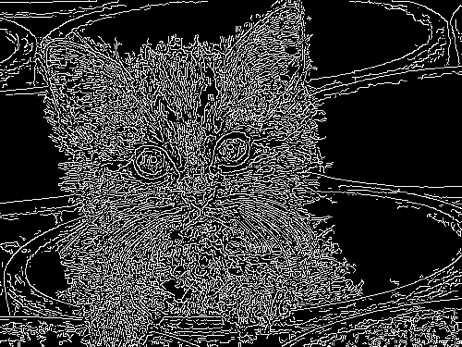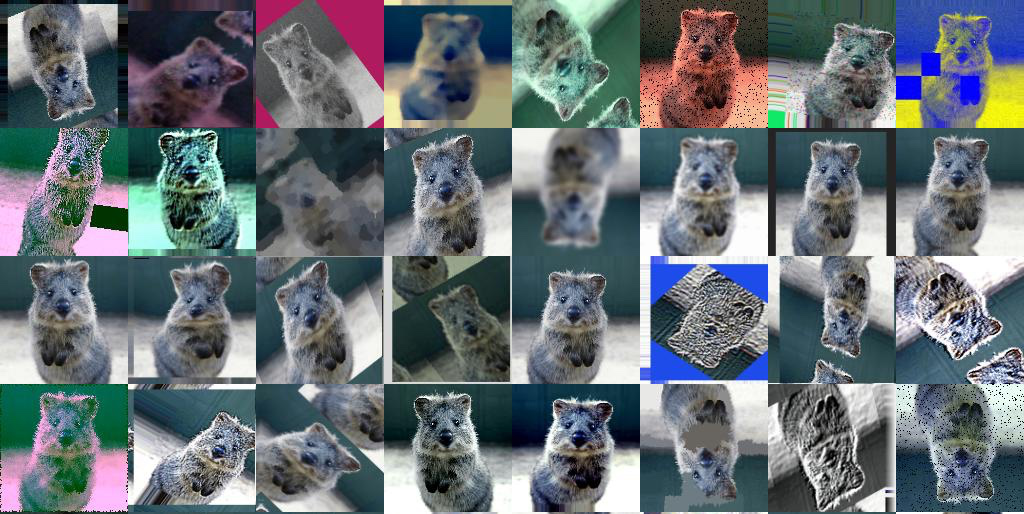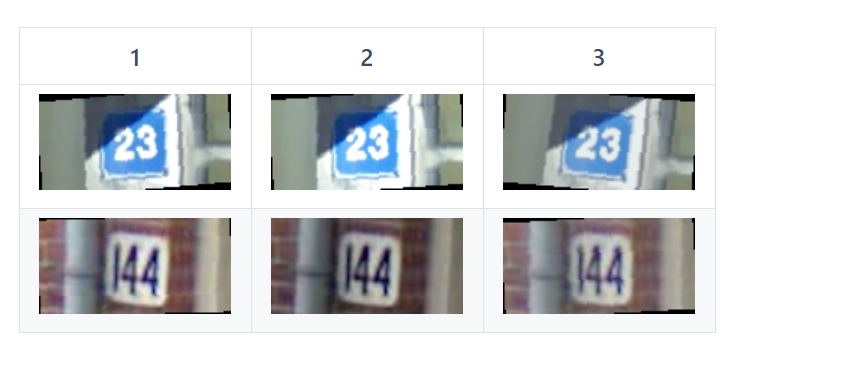# 数据读取与数据扩增

## 一.学习目标

• 学习Python和Pytorch中图像读取
• 学会扩增方法和Pytorch读取赛题数据

## 二.图像读取

### 1.Pillow

Pillow是Python图像处理函式库(PIL）的一个分支。Pillow提供了常见的图像读取和处理的操作，而且可以与ipython notebook无缝集成，是应用比较广泛的库。from PIL import Image
# 导入Pillow库

# 读取图片
im =Image.open(cat.jpg’)from PIL import Image, ImageFilter
im = Image.open(‘cat.jpg’)
# 应用模糊滤镜:
im2 = im.filter(ImageFilter.BLUR)
im2.save(‘blur.jpg’, ‘jpeg’)from PIL import Image
# 打开一个jpg图像文件，注意是当前路径:
im = Image.open(‘cat.jpg’)
im.thumbnail((w//2, h//2))
im.save(‘thumbnail.jpg’, ‘jpeg’)

### 2.OpenCV

OpenCV是一个跨平台的计算机视觉库，最早由Intel开源得来。OpenCV发展的非常早，拥有众多的计算机视觉、数字图像处理和机器视觉等功能。OpenCV在功能上比Pillow更加强大很多，学习成本也高很多。import cv2
# 导入Opencv库
# Opencv默认颜色通道顺序是BRG，转换一下
img = cv2.cvtColor(img, cv2.COLOR_BGR2RGB)import cv2
# 导入Opencv库
img = cv2.cvtColor(img, cv2.COLOR_BGR2GRAY)
# 转换为灰度图import cv2
# 导入Opencv库
img =cv2.cvtColor(img, cv2.COLOR_BGR2GRAY)
# 转换为灰度图
# Canny边缘检测
edges = cv2.Canny(img, 30, 70)
cv2.imwrite(‘canny.jpg’, edges)

OpenCV包含了众多的图像处理的功能，OpenCV包含了你能想得到的只要与图像相关的操作。此外OpenCV还内置了很多的图像特征处理算法，如关键点检测、边缘检测和直线检测等。
OpenCV官网：https://opencv.org/
OpenCV Github：https://github.com/opencv/opencv
OpenCV 扩展算法库：https://github.com/opencv/opencv_contrib

## 三.数据扩增方法

### 1.数据扩增介绍• #### 数据扩增为什么有用？### 2. 常见的数据扩增方法

• transforms.CenterCrop 对图片中心进行裁剪
• transforms.ColorJitter 对图像颜色的对比度、饱和度和零度进行变换
• transforms.FiveCrop 对图像四个角和中心进行裁剪得到五分图像
• transforms.Grayscale 对图像进行灰度变换
• transforms.RandomAffine 随机仿射变换
• transforms.RandomCrop 随机区域裁剪
• transforms.RandomHorizontalFlip 随机水平翻转
• transforms.RandomRotation 随机旋转
• transforms.RandomVerticalFlip 随机垂直翻转### 3.常用的数据扩增库

• #### torchvision

https://github.com/pytorch/vision
pytorch官方提供的数据扩增库，提供了基本的数据数据扩增方法，可以无缝与torch进行集成；但数据扩增方法种类较少，且速度中等；

• #### imgaug

https://github.com/aleju/imgaug
imgaug是常用的第三方数据扩增库，提供了多样的数据扩增方法，且组合起来非常方便，速度较快；

## 四.Pytorch读取数据

import os, sys, glob, shutil, json
import cv2

from PIL import Image
import numpy as np

import torch
from torch.utils.data.dataset import Dataset
import torchvision.transforms as transforms

class SVHNDataset(Dataset):
def __init__(self, img_path, img_label, transform=None):
self.img_path = img_path
self.img_label = img_label
if transform is not None:
self.transform = transform
else:
self.transform = None

def __getitem__(self, index):
img = Image.open(self.img_path[index]).convert('RGB')

if self.transform is not None:
img = self.transform(img)

# 原始SVHN中类别10为数字0
lbl = np.array(self.img_label[index], dtype=np.int)
lbl = list(lbl)  + (5 - len(lbl)) * 

return img, torch.from_numpy(np.array(lbl[:5]))

def __len__(self):
return len(self.img_path)

train_path = glob.glob('../input/train/*.png')
train_path.sort()
train_label = [train_json[x]['label'] for x in train_json]

data = SVHNDataset(train_path, train_label,
transforms.Compose([
# 缩放到固定尺寸
transforms.Resize((64, 128)),

# 随机颜色变换
transforms.ColorJitter(0.2, 0.2, 0.2),

# 加入随机旋转
transforms.RandomRotation(5),

# 将图片转换为pytorch 的tesntor
# transforms.ToTensor(),

# 对图像像素进行归一化
# transforms.Normalize([0.485,0.456,0.406],[0.229,0.224,0.225])
]))• Dataset：对数据集的封装，提供索引方式的对数据样本进行读取
• DataLoder：对Dataset进行封装，提供批量读取的迭代读取

import os, sys, glob, shutil, json
import cv2

from PIL import Image
import numpy as np

import torch
from torch.utils.data.dataset import Dataset
import torchvision.transforms as transforms

class SVHNDataset(Dataset):
def __init__(self, img_path, img_label, transform=None):
self.img_path = img_path
self.img_label = img_label
if transform is not None:
self.transform = transform
else:
self.transform = None

def __getitem__(self, index):
img = Image.open(self.img_path[index]).convert('RGB')

if self.transform is not None:
img = self.transform(img)

# 原始SVHN中类别10为数字0
lbl = np.array(self.img_label[index], dtype=np.int)
lbl = list(lbl)  + (5 - len(lbl)) * 

return img, torch.from_numpy(np.array(lbl[:5]))

def __len__(self):
return len(self.img_path)

train_path = glob.glob('../input/train/*.png')
train_path.sort()
train_label = [train_json[x]['label'] for x in train_json]

SVHNDataset(train_path, train_label,
transforms.Compose([
transforms.Resize((64, 128)),
transforms.ColorJitter(0.3, 0.3, 0.2),
transforms.RandomRotation(5),
transforms.ToTensor(),
transforms.Normalize([0.485, 0.456, 0.406], [0.229, 0.224, 0.225])
])),
batch_size=10, # 每批样本个数
shuffle=False, # 是否打乱顺序
num_workers=10, # 读取的线程个数
)

break


torch.Size([10, 3, 64, 128]), torch.Size([10, 6])

### 1.代码补充解释

glob

• glob.glob()
用它可以查找符合特定规则的文件路径名，并将其以list的形式返回
• glob.iglob()
获取一个可编历对象，使用它可以逐个获取匹配的文件路径名。
可参考：https://blog.csdn.net/GeorgeAI/article/details/81035422

shutil

• 这是python中一个用于对文件进行移动 复制 打包 压缩 解压的模块
具体操作可参考：shutil模块详解

json

• JSON(JavaScript Object Notation) 是一种轻量级的数据交换格式，易于人阅读和编写。
函数 描述
• json.dumps 将 Python 对象编码成 JSON 字符串
• json.loads 将已编码的 JSON 字符串解码为 Python 对象
• json.dump： 是将dict类型转换为json格式字符串，存入文件
可参考：https://www.cnblogs.com/bigtreei/p/10466518.html

## 参考05-2094

#### 零基础入门CV赛事（一）

05-18238

05-2043

05-2128

05-2371

05-21144

05-2546

05-1975

05-2025

05-2232

05-2044

05-19117

#### 最新微信小程序源码

05-18179

#### 微信小程序源码-合集1

05-2081

05-2549

05-19103

05-2324

05-2636

#### 零基础入门CV赛事-字符识别模型©️2019 CSDN 皮肤主题: 创作都市 设计师: CSDN官方博客点击重新获取扫码支付